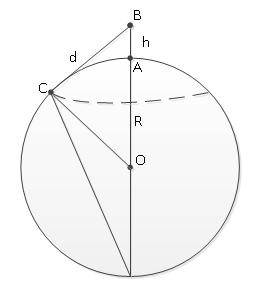# Prospect distance from the ground and line of sight distance between two observers

Calculates the prospect distance from height at observer and line of sight distance between two observers.This content is licensed under Creative Commons Attribution/Share-Alike License 3.0 (Unported). That means you may freely redistribute or modify this content under the same license conditions and must attribute the original author by placing a hyperlink from your site to this work https://planetcalc.com/1198/. Also, please do not modify any references to the original work (if any) contained in this content.

Calculator below computes the prospect distance from height at observer and line of sight distance between two observers. Some theory is placed below the calculator.#### Prospect distance from the ground and line of sight distance between two observers

Observer height in meters
Observable object height in meters
Digits after the decimal point: 3
Prospect distance

Line of sight distanceProspect distance
As shown on piture, observer's view is limited by horizon - imaginary line where sky touches the earth. According to picture we can calculate distance to the horizon from Pythagoras theorem.

where R - is Earth radius, usually taken as 6378 kilometers.
Atmospheric refraction increases the prospect distance by over 6 percent.
The result formula is

Simplified formula, which can be deduced from above, is often used
, for nautical miles or
, for kilometersLine of sight
As shown on picture, line of sight distance is simply addition of two prospect distances.PLANETCALC, Prospect distance from the ground and line of sight distance between two observers